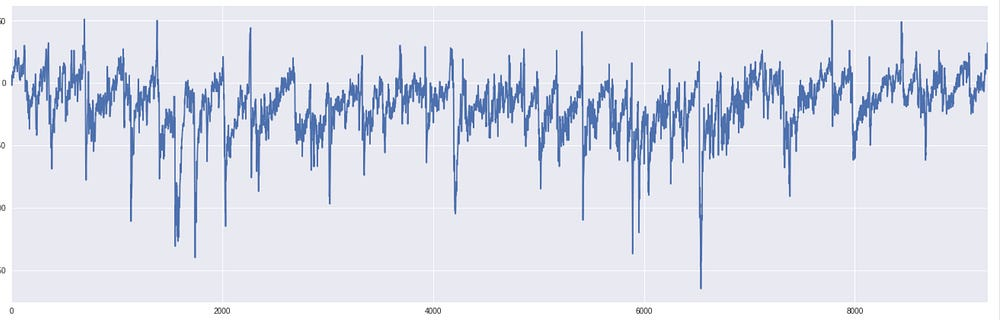Time Series Forecasting Using Empirical Mode Decomposition and (Dilated) Convolutional Networks —…

As explained in previous posts, this series is about using a method called Empirical Mode Decomposition — which is form of adaptive data analysis that doesn’t assume much about the series a priori — and decomposes the time series or signal into various orthogonal components, each at a different frequency level. In other words, it is a form of spectral analysis, and the resulting functions are called Intrinsic Mode Functions, while what is left at the end of the process, is a monotonic function — the trend.

Since this is an additive decomposition, you can reconstruct the signal by adding the parts together. And that’s what I have done here — used lagged value of a time series to predict the (decomposed) values, then added them u together. In case your’e wondering why I don’t just predict the value itself — this is because from what I can tell, the added information from the IMFs is useful, and the network learns how to weight the IMFs in the prediction of the output. It seems better than just using the time series alone.

In the following experiment, I used a time series from the physical world — magnetic field measurements — which, after separating out the last 1000 values for testing purposes, looks like this :

Here are some of the first few IMFs I get after doing the EMD process:

And here are some of the last few, including the residue at the very end.

Here is the architecture I used — this is Keras w/ Tensorflow backend:

There are just 2 conv layers in this one, both of which are concatenated with the original input, and then predicted using a conv layer that outputs a 12-tuple, since there are 12 components — 11 IMFs and a residue — that we are using as features. After training this model for 20 epochs, I tested out the predictive performance on the remaining 1000 points, and used the R2 score to get a sense of how good this fit was. Here are the results:

The R2 score I get here is about 95.6%, and the fit, in a very non-mathematical way, “looks good”.

You can see the whole notebook right here.

Source: Deep Learning on Medium